## Thursday, April 14, 2016

### Structure from Motion - Tombs and memorials (2)

Here are some 3D scene reconstructions that were obtained with Structure from Motion 10 (SfM10). The frames were extracted from short movies taken by my iphone 4 (resolution=1920x1080). To extract the frames from the videos, I used avidemux, but anything else can be used like VLC.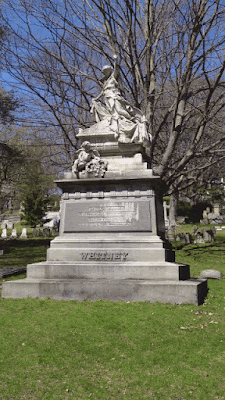Input to SfM10: 7 frames at a resolution of 1920x1080 using a focal length equal to 1920x35/36=1866.Sparse 3D scene reconstructed by SfM10 (number of trials in AC-RANSAC = 1000, max number of iterations in Bundle Adjustment = 1000, min separation angle = 1.5, and max reprojection error = 16).

Hmmm, something went terribly wrong here. Let's hike up the number of trials in AC-RANSAC and see if that's enough to solve the problem.Sparse 3D scene reconstructed by SfM10 (number of trials in AC-RANSAC = 10000, max number of iterations in Bundle Adjustment = 1000, min separation angle = 1.5, and max reprojection error = 16).

Looks like it solved the problem nicely.Input to SfM10: 7 frames at a resolution of 1920x1080 using a focal length equal to 1920x35/36=1866.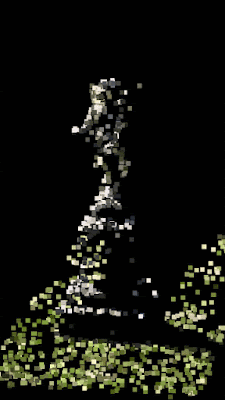Sparse 3D scene reconstructed by SfM10 (number of trials in AC-RANSAC = 1000, max number of iterations in Bundle Adjustment = 1000, min separation angle = 1.5, and max reprojection error = 16).Input to SfM10: 6 frames at a resolution of 1920x1080 using a focal length equal to 1920x35/36=1866.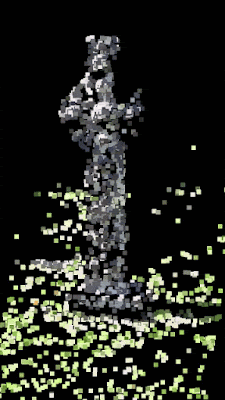Sparse 3D scene reconstructed by SfM10 (number of trials in AC-RANSAC = 1000, max number of iterations in Bundle Adjustment = 1000, min separation angle = 1.5, and max reprojection error = 16).Input to SfM10: 5 frames at a resolution of 1920x1080 using a focal length equal to 1920x35/36=1866.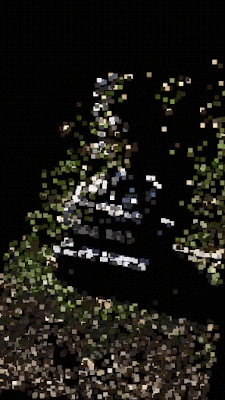Sparse 3D scene reconstructed by SfM10 (number of trials in AC-RANSAC = 1000, max number of iterations in Bundle Adjustment = 1000, min separation angle = 1.5, and max reprojection error = 16).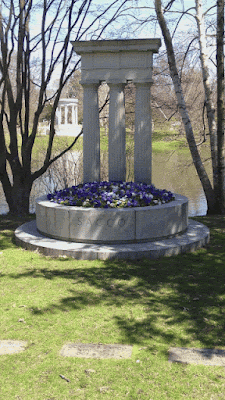Input to SfM10: 8 frames at a resolution of 1920x1080 using a focal length equal to 1920x35/36=1866.Sparse 3D scene reconstructed by SfM10 (number of trials in AC-RANSAC = 1000, max number of iterations in Bundle Adjustment = 1000, min separation angle = 1.5, and max reprojection error = 16).

Recall that the nvm file that SfM10 outputs can be used to generate a dense depth map (Multi View Stereo) with Depth Map Automatic Generator 8 or Depth Map Automatic Generator 8b (DMAG8b.